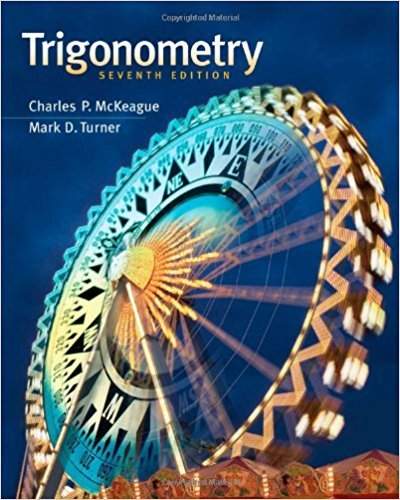×
×

# If a point is rotating with uniform circular motion on aISBN: 9781111826857 186

## Solution for problem 8 Chapter 1-15

Trigonometry | 7th Edition

• Textbook Solutions
• 2901 Step-by-step solutions solved by professors and subject experts
• Get 24/7 help from StudySoup virtual teaching assistantsTrigonometry | 7th Edition

4 5 1 235 Reviews
22
3
Problem 8

If a point is rotating with uniform circular motion on a circle of radius 1 foot, find v if the point rotates at 10 revolutions per minute. Give your answer in exact form.

Step-by-Step Solution:
Step 1 of 3
Step 2 of 3

Step 3 of 3

##### ISBN: 9781111826857

The full step-by-step solution to problem: 8 from chapter: 1-15 was answered by , our top Math solution expert on 12/27/17, 07:46PM. Since the solution to 8 from 1-15 chapter was answered, more than 224 students have viewed the full step-by-step answer. This full solution covers the following key subjects: . This expansive textbook survival guide covers 85 chapters, and 4184 solutions. This textbook survival guide was created for the textbook: Trigonometry, edition: 7. Trigonometry was written by and is associated to the ISBN: 9781111826857. The answer to “If a point is rotating with uniform circular motion on a circle of radius 1 foot, find v if the point rotates at 10 revolutions per minute. Give your answer in exact form.” is broken down into a number of easy to follow steps, and 33 words.

Unlock Textbook Solution